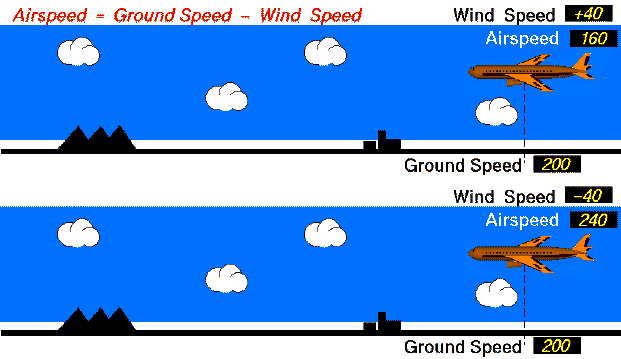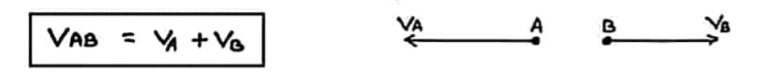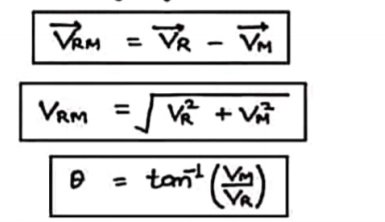Relative Velocity⁕ ﻿Relative Velocity

↪ When two objects A and B are moving with different velocities, then the velocity of one object with respect to another object is called relative velocity.↪ Here VAB is the relative velocity of object A w.r.t. B
and VBA is the relative velocity of object B w.r.t. A

↪ If both the objects are moving in same direction↪ If the two objects are moving in opposite direction↪ If the two objects are moving in opposite direction↪ If the two objects are moving at any angle θ⁕ Relative Velocity of Rain

↪ If rain is falling vertically with a velocity VR and an observer is moving horizontally with velocity VM,
↪ then velocity of rain with respect to observer will be,

or,↪ so in order to save himself from wetting person should hold umbrella at angle θ with vertical.⁕ ﻿Swimming into the river

↪ ﻿A man can swim with velocity V i.e. it is the velocity of man w.r.t. still water.

↪ If water is also flowing velocity VR then velocity of man relative to ground is,↪ If the swimming is in the direction of flow of water then↪ If the swimming is in opposite direction to the flow of water then,⁕ For Shortest path↪ If man wants to cross the river such that his displacement should be minimum then is means that he wants to reach just opposite point across the river.

↪ Man should start swimming at an angle θ with the perpendicular to flow of the river.

↪ So that its resultant velocity
VM = V + VR is in the direction of displacement of AB.

↪ To reach at B,⁕ For minimum time↪ To cross the river in minimum time the velocity along AB (VCosθ) should be maximum.
↪ It is possible if θ = 0, i.e. swimming should start perpendicular to water current.

↪ Due to effect of river velocity i.e. his displacement will not be minimum but time taken to cross the river will be minimum.↪ In time tmin swimmer travels distance BC along the river with the speed of river VR.↪ Distance travelled along river flow = drift of man = tmin VR